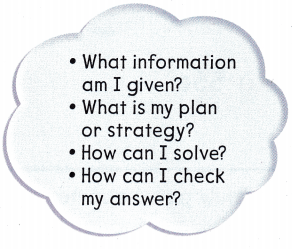Refer to our Texas Go Math Grade 1 Answer Key Pdf to score good marks in the exams. Test yourself by practicing the problems from Texas Go Math Grade 1 Unit 6 Assessment Answer Key.

Vocabulary

Use the words in the box to complete the sentences, (pp.718, 724, 736)

Charity
spend
income
needs

Question 1.
Money you earn when you work is __________.
Money you earn where you work is income.

Question 2.
Giving to others in need is __________.
Giving to others in need is charity.

Question 3.
Things that are necessary are ___________.
Things that are necessary are need.

Concepts and Skills

Question 4.
Raul’s family uses some of their income to buy services. Draw to show a service they might need and a service they might want. (TEKS 1.9.B)

Question 5.
Piper has 60¢. She buys a ball for 40¢ and a kite with the rest of her money.
Lexi has 60¢. She puts 35¢ in her bear bank and the rest in her turtle bank. (TEKS 1.9.C)
Which child saved her money? _________
Which child spent her money? __________
Write or draw to explain how you know.
Given that
Piper has 60¢.
She buys a ball for 40 ¢.
And she buys a kite with remaining money.
Lexi has 60¢
She puts 35¢ in her bear bank and rest in her trunk bank.
The child Lexi saves her money.
Child Piper spends her money.

Choose the correct answer. You may use coins.

Question 6.
Carter earns 13¢ each time he feeds the cat What is Carter’s income if he feeds the cat 3 times? (TEKS 1.9.A)
(A) 43¢
(B) 39¢
(C) 16¢
Given that
Carter earns 13¢ each time he feeds the cat.
He feeds the cat 3 times.
He earn for feeding the cat 3 times = 3 × 13 = 39 ¢.

Question 7.
Darien has 18¢. He spends 9¢ to buy a book. How much money does Darien have left? (TEKS 1.9.C)
(A) 9¢
(B) 27¢
(C) 11¢
Given that
Darien has 18¢.
He spends 9¢ to buy a book.
Darien left the money after buying a book = 18 – 9 = 9¢.

Question 8.Grant is getting dressed for school. What is the total cost of the two things above that he might need? (TEKS 1.9.B)
(A) 22¢
(B) 65¢
(C) 57¢
Given that
The cost of shirt is 7¢.
The cost of shoes is 50¢.
The total cost of two things is  7 + 50 = 57¢
Option C is the correct answer.

Choose the correct answer. You may use coins.

Question 9.
Lola puts these coins in the sharing jar. What is the total value of these coins? (TEKS 1.9.D)(A) 55¢
(B) 90¢
(C) 80¢
Lola puts these coins in the Sharing jar.
The value of the coins are 1 quarter + 1 quarter + 1 dime + 1 dime + 1 nickel + 5 cents.
1 quarter = 25 ¢
1 dime = 10 ¢
1 nickel = 5 ¢
The total value of coins are 25 ¢ + 25¢ + 10 ¢ + 10¢ + 5 ¢ + 5 ¢ = 80 cents.
Option C is the correct answer.

Question 10.
Emily puts I quarter, 3 climes, and I nickel in the sharing jar. What is the total value of these coins? (TEKS 1.9.D)
(A) 60¢
(B) 45¢
(C) 65¢
Given that
Emily puts 1 quarter, 3 dimes, 1 nickel in the sharing jar.
The total value of these coins are
1 quarter = 25 cents
1 dime = 10 cents then 3 dimes = 30 cents
1 nickel = 5 cents
The total value of these coins = 25 cents + 30 cents + 5 cents = 60 cents.
Option A is the correct answer.

Question 11.
Kari earns 15¢ each time she makes her bed. What is Kari’s income if she makes her bed 5 times? (TEKS 1.9.A)
(A) 50¢
(B) 75¢
(C) 20¢
Given that
Kari earns 15 cents each time she makes her bed.
Kari earns if she earn for makes her bed 5 Times = 5 × 15 = 75 cents.
Option B is the correct answer.

Choose the correct answer. You may use coins.

Question 12.
Sam saves 120 each week for four weeks. How much money does Sam save? (TEKS 1.9.C )
(A) 40¢
(B) 48¢
(C) 16¢
Sam saves 120 each weeks.
For four weeks she earn = 120 × 4 = 480
The total money Sam save = 480 = 48 cents.
Option B is the correct answer.

Question 13.
Betsy earns 6 coins for walking the dog. Only one coin is a quarter.
What coins could Betsy have? What is the total value?
Use numbers, pictures, or words to show your work.